# An Introduction to Atomic Number, Isotopes and Isobars

The discovery of subatomic particles provided a lot of information about the properties of the chemical elements and their isotopes. Moseley gave the hypothesis that the modern periodic table proposed by Mendeleev was based on the number of protons in the nucleus of an atom. Moseley’s hypothesis was based on the study of wavelengths of X-rays that were emitted by chemical elements. It was this hypothesis that laid the foundation for the atomic number.

## Atomic number and Mass number

### Atomic Number

The number of protons present in the nucleus of an atom is called the atomic number. It is generally represented by Z. It has been established that the charge of a proton is equal but opposite to that of an electron. Since an atom is electrically neutral and neutrons carry no electrical charge, the number of electrons and protons in a neutral atom is the same. This implies that the atomic number is equal to the number of protons or the number of electrons in an atom. As we know that elements tend to lose or gain electrons during many chemical reactions, the number of protons is conventionally used to represent the atomic number of an element. Hydrogen has the atomic number of one as it has only one proton in its atom.

Atomic Number (Z) = Number of protons in the nucleus of an atom

Example : Carbon atomic Number is 6 and it has 6 number of Protons

### Mass Number

It is the total number of protons and neutrons present in the nucleus of an atom. Protons and neutrons present in the nucleus of an atom are together called nucleons and hence the mass number is the number of nucleons present in an atom. The mass number is represented by A. Example: Nitrogen has a mass number of 14 as it has 7 protons and 7 neutrons in its atom.

Mass Number (A) = Number of Protons + Number of neutrons

Example: Carbon atom Number of Protons =6 , Number of number of Neutrons= 6,

Mass Number =12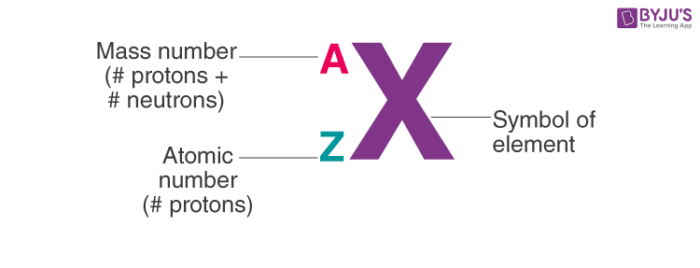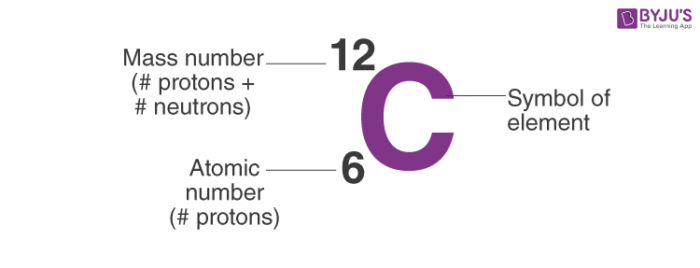## Isotopes and Isobars

### Isotopes

Those elements that have the same atomic number but a different mass number are referred to as isotopes. Isotopes occur due to the presence of a different number of neutrons in elements having the same atomic number as mass number is the sum of the number of neutrons and protons. Many but not all elements have isotopes. The isotopes of hydrogen are protium (has one proton and no neutrons), deuterium (has one proton and one neutron) and tritium (has one proton and two neutrons). The chemical properties of isotopes are the same owing to the fact that they have the same number of protons and hence the same number of electrons which determines the chemical properties of an element.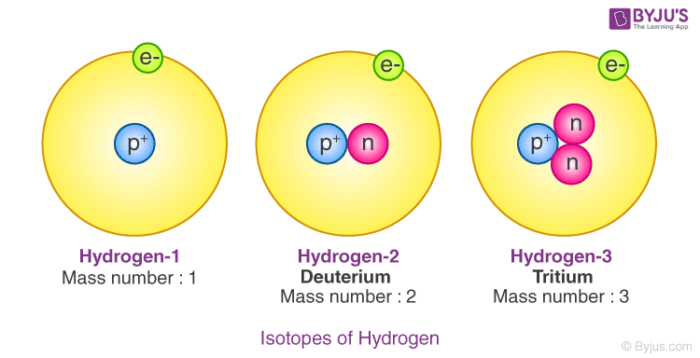### Isobars

Isobars, on the other hand, are the atoms having the same mass number but a different atomic number. For example, the atomic number of Argon is 18 and mass number is 40, the atomic number of Potassium is 19 and the mass number is 40 similarly the atomic number of Calcium is 20 and mass number is 40. Argon, Potassium and Calcium are the same mass numbers but different atomic numbers so they are Isobars with each other. Similarly the atomic number of carbon and nitrogen is 6 and 7 respectively. Carbon-14 an isotope of carbon has a mass number of 14 which is same as that of nitrogen and hence carbon-14 and nitrogen are isobars.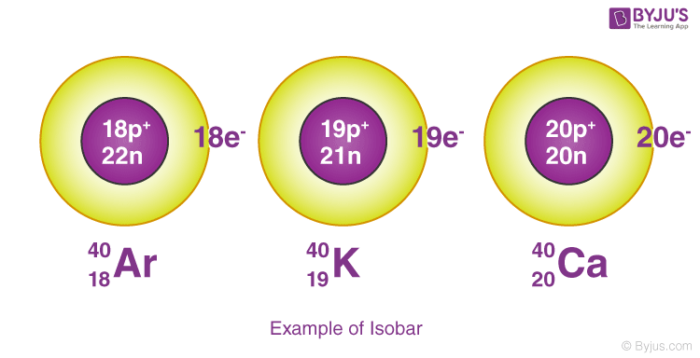## Difference Between Isotopes and Isobars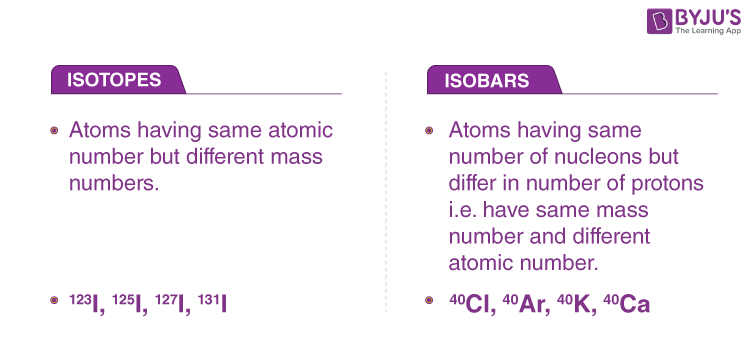Atomic numbers play a very important role in the placement of elements in the modern periodic table. It represents the number of protons and hence the number of electrons in an atom. The number of electrons in an atom governs its chemical behaviour. The study of elements has revealed their applicability in different forms. Learn more about these elements with the expert faculty at BYJU’S.

## Frequently Asked Questions – FAQs

Q1

### What is isotopes and isobars with examples?

Those elements that have the same atomic number but a different mass number are referred to as isotopes. On the other hand, Isobars are the atoms having the same mass number but a different atomic number. Examples: The isotopes of hydrogen are protium (has one proton and no neutrons), deuterium (has one proton and one neutron) and tritium (has one proton and two neutrons). Argon, Potassium and Calcium are the same mass numbers but different atomic numbers so they are Isobars with each other

Q2

### What is atomic number and isotopes?

The number of protons present in the nucleus of an atom is called the atomic number, whereas the Isotope is the elements that have the same atomic number but a different mass number.

Q3

### Is isotopes the same as atomic numbers?

The Isotopes of elements are the same atomic number or same proton but different neutron. So all Isotopes have the same atomic number. Example hydrogen show three Isotopes i.e protium (has one proton and no neutrons), deuterium (has one proton and one neutron) and tritium (has one proton and two neutrons)

Q4

### Which particle has the greatest mass?

Atoms have three fundamental particles i.e proton, electron and neutron. Among them, the neutron is the heaviest one.

Q5

### Why do isotopes occur?

Most Isotopes are formed naturally on the spontaneous process through radioactive decay of a nucleus or some are formed artificially by bombarding a stable nucleus with charged particles via accelerators or neutrons in a nuclear reactor.

Test Your Knowledge On An Introduction To Atomic Number Isotopes And Isobars!